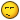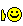# Linear Programing

5 replies to this topic

### #1Frank

Frank

Newbie

•• Members
•• 3 posts
• Location:Portugal

• Calculators:

Posted 02 December 2004 - 05:44 PMCan anyone tell me where can i found a program to solve Linear Programing??? I can found eActivity file, but i was anable to found a program to solve it...### #2Guest_Guest_*

Guest_Guest_*
•• Guests

Posted 04 December 2004 - 01:21 AMCan anyone tell me where can i found a program to solve Linear Programing??? I can found eActivity file, but i was anable to found a program to solve it...<{POST_SNAPBACK}>

sorry, what is linear programing???

### #3Frank

Frank

Newbie

•• Members
•• 3 posts
• Location:Portugal

• Calculators:

Posted 06 December 2004 - 03:20 PM

Q1. "What is Linear Programming?"

A: (For rigorous definitions and theory, which are beyond the scope of this document, the interested reader is referred to the many LP textbooks in print, a few of which are listed in the references section.)

A Linear Program (LP) is a problem that can be expressed as follows (the so-called Standard Form):

minimize cx
subject to Ax = b
x >= 0

where x is the vector of variables to be solved for, A is a matrix of known coefficients, and c and b are vectors of known coefficients. The expression "cx" is called the objective function, and the equations "Ax=b" are called the constraints. All these entities must have consistent dimensions, of course, and you can add "transpose" symbols to taste. The matrix A is generally not square, hence you don't solve an LP by just inverting A. Usually A has more columns than rows, and Ax=b is therefore quite likely to be under-determined, leaving great latitude in the choice of x with which to minimize cx.
The word "Programming" is used here in the sense of "planning"; the necessary relationship to computer programming was incidental to the choice of name. Hence the phrase "LP program" to refer to a piece of software is not a redundancy, although I tend to use the term "code" instead of "program" to avoid the possible ambiguity.

Although all linear programs can be put into the Standard Form, in practice it may not be necessary to do so. For example, although the Standard Form requires all variables to be non-negative, most good LP software allows general bounds l <= x <= u, where l and u are vectors of known lower and upper bounds. Individual elements of these bounds vectors can even be infinity and/or minus-infinity. This allows a variable to be without an explicit upper or lower bound, although of course the constraints in the A-matrix will need to put implied limits on the variable or else the problem may have no finite solution. Similarly, good software allows b1 <= Ax <= b2 for arbitrary b1, b2; the user need not hide inequality constraints by the inclusion of explicit "slack" variables, nor write Ax >= b1 and Ax <= b2 as two separate constraints. Also, LP software can handle maximization problems just as easily as minimization (in effect, the vector c is just multiplied by -1).

The importance of linear programming derives in part from its many applications (see further below) and in part from the existence of good general-purpose techniques for finding optimal solutions. These techniques take as input only an LP in the above Standard Form, and determine a solution without reference to any information concerning the LP's origins or special structure. They are fast and reliable over a substantial range of problem sizes and applications.

Two families of solution techniques are in wide use today. Both visit a progressively improving series of trial solutions, until a solution is reached that satisfies the conditions for an optimum. Simplex methods, introduced by Dantzig about 50 years ago, visit "basic" solutions computed by fixing enough of the variables at their bounds to reduce the constraints Ax = b to a square system, which can be solved for unique values of the remaining variables. Basic solutions represent extreme boundary points of the feasible region defined by Ax = b, x >= 0, and the simplex method can be viewed as moving from one such point to another along the edges of the boundary. Barrier or interior-point methods, by contrast, visit points within the interior of the feasible region. These methods derive from techniques for nonlinear programming that were developed and popularized in the 1960s by Fiacco and McCormick, but their application to linear programming dates back only to Karmarkar's innovative analysis in 1984.This is linear programing... was i boring, sorry!But what i need, is a program that solves Simplex method, above described. thanks to u all.### #4coco

coco

Newbie

•• Members
•• 23 posts

Posted 07 December 2004 - 08:40 PM

Hi, follow this link and look for Alan Ziv's programs. The one called LINPROG is what you are looking for, unfortunateley it is only suitable for CFX and AFX but I think the porting to CP300 basic must not be so hard to do. Hope it helps

http://www.charliewa...io/casprog.html

### #5manuelfrank12

manuelfrank12

Casio Fan

•• Members
•• 34 posts

• Calculators:

Posted 08 December 2004 - 01:14 AM

good explanation
thanks

### #6Frank

Frank

Newbie

•• Members
•• 3 posts
• Location:Portugal

• Calculators:
Thank's coco, for your help